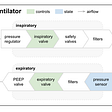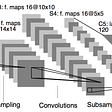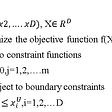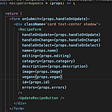# Images are Numpy arrays

A is a grid of values, all of the same type. These values contain information about each pixel of the image. It is the primary information stored in the pixels and determines the intensity of light from each point of the image. Because images are Numpy arrays, arithmetic operations can be performed on them like any other array.

To have a hands-on-experience as you read, I recommend you install these python libraries: NumPy, Matplotlib, Scipy, and Scikit-image.

Let’s start by generating a Numpy array of random integers.

Code Summary

• np.random.seed(0): This function generates the same random numbers every time from the bitGenerator if fed with the same argument. The objective of the code is to ensure the reproducibility of the generated random matrix.
• np.set_printoptions(threshold=sys.maxsize): This function determines the way arrays and other Numpy objects are displayed. The objective of the code is to print all the values of the Numpy array. The default setting truncates the printed array.
• np.random.randint(0, 50, (15,15)): This function returns random integers from “low” (inclusive) to “high” (exclusive). The objective of the code is to return random integers from 0(inclusive) to 50(exclusive), with 15 rows and 15 columns.

Let’s see how the generated Numpy arrays look as an Image.

Figure 2. shows the image representation of the generated Numpy matrix in figure 1. It can be observed that the pixels in the image show different shades of black and white. This observation is a function of the magnitudes of the values of the array. The higher the value, the “whiter” it is. Likewise, the lower the value, the “darker” it becomes.

A popular convention for representing image values is a range from 0 -1; with zero (0) being black, one (1) being white, and the values between representing the different shades between black and white. There is the 0–255 convention. Here also, 0 represents black and 255 represents white with the range representing the shades between black and white.

Let’s perform an operation on the generated arrays and see how it will transform the image.

First, we will slice off a portion of the center of the generated array in fig.1, then, we will replace the sliced portion with the lowest value in the array, which is zero, and then observe the transformation.

Code Summary

• image_array[5:10, 5:10] = 0; This code sliced the image array from the fifth row to the tenth row, and from the fifth column to the tenth column. Then, it replaced all the values in the sliced portion with zero.

Since zero maps to black in the image value convention, it produced black pixels at the the sliced portion.

Let’s perform another slicing operation, this time, replacing with 255, and then, observe the image transformation. Can you guess what we ought to see?

As you may have guessed right, replacing the sliced portion with 255 produces white pixels in that area.

If you compare the images in fig. 3 and fig. 4, you will realize the area around the sliced portion of the image in fig. 4 appears darker than the image in fig. 3, even though the values in those areas were not touched.

This effect is due to the sharp or steep pixel intensity variation between the white pixels (255) at the sliced portion and the surrounding pixels in fig. 4. 255 is farther away on the number line compared to the values of the surrounding pixels. Thus, the sharp variation accentuates the intensity of the white pixels, thereby making the surrounding pixels appear darker. Fig 3 didn’t produce this effect because of the same concept. In that case, the values of the sliced portion (zero) are closer in magnitude to the values of the surrounding pixels. As a result, the pixels emit similar intensities of light, thus, giving the ability to see each pixel as being distinct.

# Filters are operations performed on images.

Just as we sliced a portion of the image array to produce certain color effects, filters are produced in like manner. Filters can be used to blur images, sharpen images, detect the edges in images, and several others. Basically, filters enhance features in images and can also reduce noise in them.

Henceforth, when you blur an image on your mobile phone, or you use a filter on Snapchat, realize that you’ve performed an arithmetic operation on your image arrays.

There are two key concepts that we need to understand in other to grasp filters in image processing; Kernels and Convolution.

# Kernels

Kernels are matrices used to produce effects (blurring, sharpening, outlining) in images. They are mostly 2-dimensional arrays and are often used interchangeably with filters. There are 1-dimensional kernels, 3-dimensional kernels, etc. In 3D however, you are likely to hear more of filters than kernels.

The dimension of the kernel and the image to be convolved with must be the same. A 1D kernel can convolve with a 1D image (signal), a 2D kernel convolves with a 2D image. Likewise, a 3D filter (group of kernels) convolves with a 3D image.

Some examples of kernels:

# Convolution

Convolution is a mathematical operation that multiplies two arrays of the same dimensionality to produce a new array of the same dimensionality. This is achieved by running or sliding one of the arrays (kernel) across the other array (image array). For every pixel of the image, we slide or map the kernel over it and then multiply each pixel value of the image with the corresponding value of the kernel. Afterward, we take the sum of the product values which are used to replace pixel values of the image. Let’s illustrate;

As explained above, the kernel is slid across the image array and the sum of the products is calculated and used to replace the pixel value of the image under focus. The diction used is “The image array is convolved with the kernel” or “The kernel is convolved with the image array”.

Convolution is represented mathematically as:

Let’s demonstrate the convolution operation with a 2x2 matrix in old school way:

For clarity, when the kernel is mapped over the image matrix, only the color codes will be used and not the values. The color codes should be thought of as a representation of the values indicated in the kernel.

Manual Convolution Computation:

In the illustration above, the image matrix is padded with zeros as a way of extending the edge of the image array. The reason for extending the edge is to accommodate the values of the kernel that extend beyond the edges of the image array when it is mapped over it. There are other ways of padding or extending the edges. I encourage you to find out the effect each option would have on the resulting image matrix.

In the next illustration, we will compare our result obtained in the manual computation with Scipy’s convolution function.

Code Summary

• np.array([(10,20), (30,40)]): This function creates a Numpy array. The objective of the code is to create a 2D array with the elements, 10,20,30, and 40.
• ndi.convolve(img_matrix, kernel_matrix, mode=”constant”, cval=0): This function performs a multi-dimensional convolution. The objective of the code is to convolve the img_matrix with the kernel_matrix. The mode=” constant” pads/extends the img_matrix by filling all the values beyond the edge with the same constant value(0), defined by the “cval” parameter.

Don’t beat yourself up when you don’t get the expected output matrix as we had during the manual computation. The convolve function from Scipy returns only the original region of the new array (output_matrix). If we are to take the original region of the result from our manual computation, we will also end up with a 2x2 array, just like Scipy’s.

This supposes that Scipy also had a 4x4 matrix but returned a 2x2 matrix. With this, I think we can consider our manual computation validated.

## Mean Kernel

Code Summary

• ndi.correlate(image, kernel): Like the ndi.convolution(), ndi.correlate() performs multi-dimensional correlation. The objective of the code is to correlate the image with the kernel. If you aren’t familiar with correlation, I recommend you look it up. It shares many similarities with the convolution function.

The mean kernel has the effect of blurring images. A preferred kernel for blurring is the Gaussian filter. It is preferred because it produces a more uniform or smoother intensity distribution on the images it’s convolved with.

Let’s compare the effects of the mean and Gaussian kernels to confirm the statement above.

Code Summary

• np.full((2,2), 1/9): This function returns a new array of the given shape and type, filled with the “fill_value”. The objective of the code is to create an array of two rows(2) and two columns(2) filled with the value 1/9.
• filters.gaussian(image): This function performs multi-dimensional Gaussian filtering. The objective of the code is to apply the Gaussian filter to the image.

It can be observed in fig.13 that, the Gaussian kernel produces a “smoother” image than the mean kernel’s, hence the preferred choice when blurring images.

Let’s get a “feel” of the kernels on real images. Shall we?

## Identity Kernel

Code Summary

• color. rgb2gray(image): This function computes luminance on an RGB image. The objective of the code is to convert the color image of the Mona Lisa to a gray image. The returned image is a 2D array since the channel dimension is removed during the conversion process.

If you can see a transformation in the output image in fig. 14, then you really have some powerful eyes!

The identity kernel returns an output just as the input. That is to say, it has no effects on images.

## Edge-detection Kernel

The edge-detection kernel detects edges in images. It can be observed that the edges around the eyes, mouth, nose, and head have been detected in the output image.

## Sharpen Kernel

The Sharpen kernel gives images sharper appearances. It achieves this by increasing the contrast between the bright and dark regions of the image.

## Gaussian Kernel

The Gaussian kernel blurs images. It produces a “smoothing” effect on images.

I think the Mona Lisa in the output image looks happier. Probably, because of her “smoother” skin now.

So, I tried tweaking with the identity kernel to see if it had some hidden effects. With one of the tweaks, I replaced the integer(1) in the identity kernel with -1.

Guess what I saw!

I don’t know if this tweaked identity filter/kernel has a name or not, what I care about is that, by manipulating the arrays of images and filters/kernels, it can produce effects that can be useful in image processing. Thanks for reading!

If you would like to have more hands-on-experience with image processing, I recommend you watch the videos titled “ Image Analysis in Python with Scipy and scikit-image — Stefan Van de Walt”.

--

--

--

## More from Bernard Baah Abboah

Data Science enthusiast - Interest in image processing

Love podcasts or audiobooks? Learn on the go with our new app.

## Creating a Machine Learning Model to Predict Malignant Breast Cancer Tumors## Train anything with Lightning custom Loops## Automation of Mechanical pressure-controlled ventilator## A Sneak Peek Into ML## Part 4: Image Classification## Differential evolution : What it is and how does it work?## Starting Natural Language Processing## Bernard Baah Abboah

Data Science enthusiast - Interest in image processing

## Final Flatiron Project## How I got Into Red Hat, Part 3